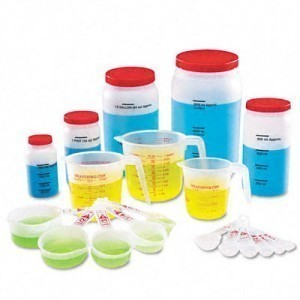# Liquid Measurements

How many liters do you need? How many teaspoons should you have? Figuring out the amounts is not that hard anymore. In fact, there are many ways to convert liquid measurements so as to cater to the standard measurements that you may need to follow.

Where shall you use those conversion units? Well, to putter in the kitchen may be the main concern when you find that you need to convert certain measurements of liquid to know the appropriate amounts needed for a certain recipe.

For your guidance, here are some ways to convert liquid measurements:

### When You Need to Convert Within the Standards of the Metric System

To learn how to convert certain amounts of liquids in accordance with the metric system, here are a few notes to bear in mind:

One: For ten milliliters, that may simply equal to about one centiliter.

Two: If you want to learn about how many deciliters would be equivalent to a liter, remember that that would take about ten deciliters (or one thousand ml) to equal that measurement.

Three: If you need to convert an ounce with a certain amount of milliliters of liquid, just bear in mind that one fluid ounce is about the same amount when you have about thirty milliliters (30 ml) or 29.573 ml.

Four: For measurements for a cup and milliliters, that would be about 236.584 ml or exactly 240 ml that would be similar to a cup’s amount of liquid.

Five: For a quart, the equivalent measurement would be about 0.94635 liters or 1 liter exactly.

Six: To convert a gallon to liters, that would take about 3.7854 liters or exactly 4 liters to have similar measurements for a gallon of liquid.

### For Measurement Conversions in Accordance to the U. S. System

One: In terms of converting certain teaspoon amounts of liquid to a tablespoon or an ounce, the serving size or amounts would be as such: A tablespoon would equal to around three teaspoons. Around 2 fluid ounces or ¼ cup of liquid would be equivalent to four tablespoons.

Two: If you want to know the equivalent of a cup to ounces that would be around eight ounces of liquid to a cup. Around two cups would be similar in measurement to a pint.

Three: To get the measurement equivalents of two pints to ounces, that would be around thirty two fluid ounces or a quart.

Four: If you need to know the equivalent measurement of four quarts to ounces that would take around 128 ounces or one gallon.

Five:
For a barrel, the equivalent measurement would be three and one half of gallons. For two barrels, that would equal to one hogshead.

These liquid measurements may be a bit confusing at first. But once you get the hang of it, you will be able to make the necessary conversions as needed.